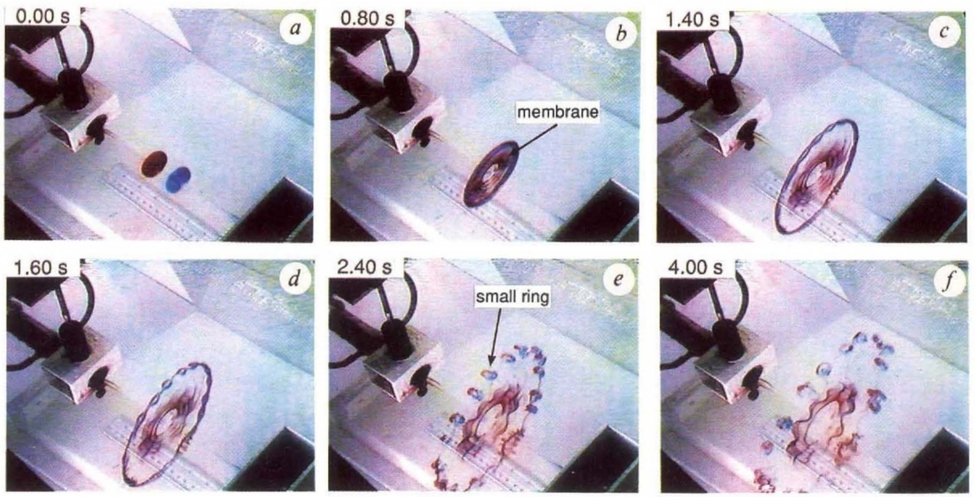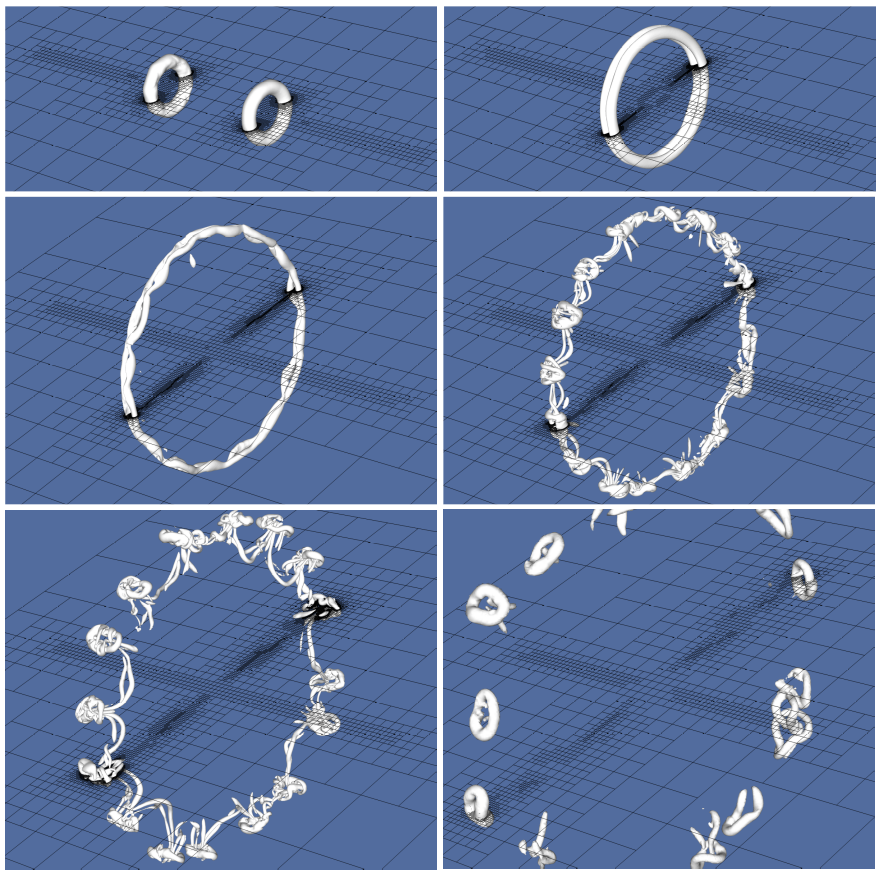# sandbox/Antoonvh/two_rings.cThe experiment of Lim and Nickels (1992).

# The collision of two vortex rings.

400000 dye particles (Video via surfdrive)

The \lambda_2 vortex-detection isosurfaces reveal the dynamics in great detail. (Video via surfdrive)

## Set-up

#include "grid/octree.h"
#include "navier-stokes/centered.h"
#include "navier-stokes/perfs.h"
#include "fractions.h"
#include "view.h"
#include "lambda2.h"
#include "tracer-particles.h"
#include "scatter2.h"

Particles red, blue;

scalar f[];
int maxlevel = 11;
double ti = 4., ue = 0.008;
double Re = 1750.;
double tend = 120. + 0.1;
int np = 2e5;

## Boundary conditions

Initially, there are two opposing jets at the left an right wall. The top and bottom boundary can leak fluid.

u.n[left]   = dirichlet( f[]  *(1.) * (t <= ti));
u.n[right]  = dirichlet(-f[-1]*(1.) * (t <= ti));
u.n[top]    = neumann (0.);
p[top]      = dirichlet (0.);
pf[top]     = dirichlet (0.);
u.n[bottom] = neumann (0.);
p[bottom]   = dirichlet (0.);
pf[bottom]  = dirichlet (0.);

int main() {
L0 = 32.;
X0 = Y0 = Z0 = -L0/2;
const face vector muc[] = {1./Re, 1./Re, 1./Re};
mu = muc;
run();
}

The simulations starts when the jets are triggered. We have a guess at an initial grid.

event init (t = 0) {
refine (RADIUS < 2.5 && fabs(x) > 9.*L0/20.   && level < (maxlevel - 1));
refine (RADIUS < 1.5 && fabs(x) > 19.5*L0/40. && level < (maxlevel));
f.refine = f.prolongation = fraction_refine;
boundary ({f});
new_tracer_particles (0); //This should not be needed
}

During the injection phase, the orifice shape is recomputed.

event inject (i++; t <= ti) {
boundary ({f});
}

## Particles

Tracer particles are added as a sort of dye. This is done right after the injection. Special care is taken to make this step MPI compatible.

event add_tp (t = ti) {
if (pid() == 0) {
red  = new_tracer_particles (np);
blue = new_tracer_particles (np);
int j = 0;
double Rseed = 1.8; //The rings are larger than the opening
while (j < np) {
double yp = noise();
double zp = noise();
if (sq(yp) + sq(zp) < sq(1.)) {
pl[red][j].x  = X0 +      fabs(noise()*ti*0.35);
pl[red][j].y  = yp*Rseed;
pl[red][j].z  = zp*Rseed;
pl[blue][j].x = X0 + L0 - fabs(noise()*ti*0.35);
pl[blue][j].y = yp*Rseed;
pl[blue][j].z = zp*Rseed;
j++;
}
}
} else {
red  = new_tracer_particles (0);
blue = new_tracer_particles (0);
}
particle_boundary (red);
particle_boundary (blue);
set_particle_attributes (red);
set_particle_attributes (blue);
}

## Output

Two movies are generated, plotting an adaptive \lambda_2-isosurface value, a slice of the the cells and the the particles.

event snapshots (t += 0.1) {
char str;
sprintf (str,"Re = %g", Re);
double val = -0.0001;
scalar l2[];
lambda2 (u, l2);
foreach()
l2[] = l2[] < val ? l2[] : nodata;
stats l2s = statsf(l2);
foreach()
l2[] = l2[] < val ? l2[] : 0;
boundary ({l2});
int w = 1280;
double tzoom = 100;
double fov = t < tzoom ? 15 - t/80 : 15 - t/80 - (t - tzoom)/2.5;
view (fov = 15 - t/80, width = w, height = 9*w/16, bg = {0.3, 0.4, 0.6},
theta = 0.6 + 0.15*cos(t/15), phi = 0.6, ty = 0.05);
isosurface ("l2", min (-l2s.stddev, val));
translate (y = -L0/4)
cells (n = {0,1,0});
draw_string (str, pos = 2, lw = 3);
save ("movl2.mp4");

if (blue) {
clear();
view (bg = {0.9, 0.9, 0.9});
scatter (red,  s = 7, pc = {0.8, 0.2, 0.2});
scatter (blue, s = 7, pc = {0.2, 0.2, 0.8});
translate (y = -L0/4)
cells (n = {0,1,0});
draw_string (str, pos = 2, lw = 3);
save ("movp.mp4");
}
}

event dumps (t += 10) {
char fn;
sprintf (fn, "dump%g", t);
dump (fn);

sprintf (fn, "red_blue%g", t);
pdump (fn);
}

adapt_wavelet ((scalar*){u}, (double[]){ue, ue, ue}, maxlevel);

event stop (t = tend);

## Results

Visualization of the \lambda_2 iso-surface reveals detail: